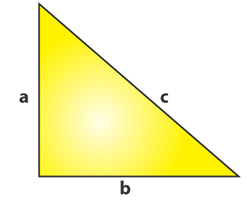# Pythagorean Theorem Word Problem Worksheets

What is the Purpose of the Pythagorean Theorem? Pythagorean theorem describes the relationship between the sides of a right-angled triangle. The Pythagorean formula is applied on a right-angled triangle and is used to determine the hypotenuse, base and the perpendicular of the triangle. The theorem states that: "In a right-angled triangle, the square of the hypotenuse side is equal to the squares of the perpendicular side and the base side." The hypotenuse is the longest side, and perpendicular is the side opposite to the hypotenuse side.Consider the triangle above, where a is the perpendicular side, b is the base side, and c is the hypotenuse. According to the definition of the Pythagorean theorem, the formula would be written as: c2 = a2 + b2. Pythagorean theorem allows us to find the length of a side when two lengths are known, making it a useful technique in navigation and construction. When we have two known sides, we can easily find out the length of the diagonal. This approach is used in construction projects, woodworking, and architecture. Also, the Pythagorean theorem is used for two-dimensional navigation. When drawing a map, cartographers use the Pythagorean theorem to find the height and distance between different points.

• ### Basic Lesson

Demonstrates how to outline Pythagorean Theorem Word Problems. Example: One leg of a triangle is 4 cm and other leg is of 3 cm. What is the hypotenuse?

• ### Intermediate Lesson

Uses slightly larger sentences and numbers than the basic lesson. Example: The hypotenuse of a triangle is 5 cm and base is of 3 cm. Find the height.

• ### Independent Practice 1

Contains a series of 20 Pythagorean Theorem Word Problems. The answers can be found below. Example: Tom wants to swim across a river that is 630 meters wide. He begins swimming perpendicular to the shore he started from but ends up 200 meters down river from where he started because of the current. How far did he actually swim from his starting point?

• ### Independent Practice 2

Features 20 Pythagorean Theorem Word Problems. Example: Town A is 8 miles from town B, and 14 miles from town C. Town A, B and C are forming a right triangle at A. A road connects towns B and C directly. Find the length of this road.

• ### Homework Worksheet

12 word problems for students to work on at home. An example problem is provided and explained. Example: An 82 feet ladder is leaned against a wall. If the base of the ladder is 80 feet from the wall, how high up the wall will the ladder reach?

• ### Skill Quiz

10 Pythagorean Theorem Word Problems. A math scoring matrix is included. Example: Dick rides his bike 68 km south and then 6 km west. How far is he from his starting point?

• ### Homework and Quiz Answer Key

Answers for the homework and quiz.